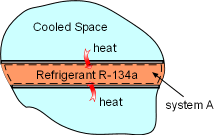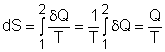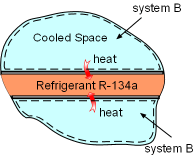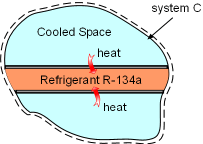Ch 6. Entropy Multimedia Engineering Thermodynamics Entropy Tds Relations EntropyChange IsentropicProcess IsentropicEfficiency EntropyBalance (1) EntropyBalance (2) ReversibleWork
 Chapter 1. Basics 2. Pure Substances 3. First Law 4. Energy Analysis 5. Second Law 6. Entropy 7. Exergy Analysis 8. Gas Power Cyc 9. Brayton Cycle 10. Rankine Cycle Appendix Basic Math Units Thermo Tables Search eBooks Dynamics Fluids Math Mechanics Statics Thermodynamics Author(s): Meirong Huang Kurt Gramoll ©Kurt GramollTHERMODYNAMICS - CASE STUDY SOLUTION

Refrigerant R-134a vaporizes in the evaporator and absorbs heat from the cooled space. Entropy changes of the Refrigerant R-134a, the cooled space and the whole process need to be determined.

 Saturated R-134a Temperature Table Saturated R-134a Pressure Table< < Superheated Steam Table

Refrigerant R-134a enters the evaporator as a saturated liquid-vapor mixture and leaves the evaporator as saturated vapor. Hence the temperature in the evaporator remains constant at the saturation temperature of 200 kPa, which is,

Tsat. @ 200 kPa = -10.09oCHeat Transferred into System A

(1) Determine the entropy change of refrigerant R-134a

Take refrigerant R-134a as a system (system A). It enters the evaporator as a saturated liquid-vapor mixture, which is state 1.

P1 = 200 kPa
T1 = Tsat. @ 200 kPa = -10.09oC

Refrigerant R-134a leaves the evaporator as saturated vapor, which is state 2.

P2 = 200 kPa
T2 = Tsat. @ 200 kPa = -10.09oC

During this process, refrigerant R-134 absorbs 150 kJ heat.

Q = 150 kJ

Hence, the entropy change for refrigerant R-134a from process 1-2 isTemperature T is a constant in this case, and the above equation can be integrated asSubstituting Q and T into the above equation yields,

ΔSA = 150(1000)/(-10.09 + 273) = 570.56 J/KHeat Transferred out of System B

(2) Determine the entropy change of the cooled space

Take the cooled space as a system (system B). During the heat transfer process, the space is maintained at -4oC and it dissipates 150 kJ heat to refrigerant R-134a. The entropy change of the cooled space can be determined as

ΔSB =Q/T = -150(1000)/(-4 + 273) = -557.6 J/KSystem C is an Isolated System

(3) Determine the total entropy change of the process

Take refrigerant R-134a and the cooled space together as a system (system C). Then refrigerant R-134a and the cooled space are subsystems of system C. The entropy change of system C is the sum of the entropy changes of its two subsystems.

ΔSC = ΔSA+ ΔSB
= 570.56 + (-557.6) = 12.44 J/K

System C is an isolated system since no heat and mass transfer out of its boundaries. The entropy change of system C is greater than 0, which satisfies the increase of entropy principle.